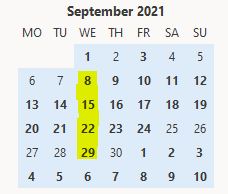# Dates - How to get the nth weekday for a month

When building apps, it can be very useful to determine the nth workday in a month. For example, what's the 2nd Tuesday in a month, what's the 3rd Wednesday for a month, what's the first Monday (etc)? This post describes the formula to carry out this task.

It can be very useful to determine the nth workday in a month, particularly for scheduling apps, where we may want to create reoccurring events for a given day in a month.

## Generic Formula - Example - How to get the 2nd Tuesday for a given month

To demonstrate how to perform this calculation, the formula to get the second Tuesday for September 2021 looks like this:
`With({FirstOfMonth:Date(2021,9,1),      DayOfWeek:3,      N:2     },     DateAdd(FirstOfMonth,             (N * 7) - Weekday(DateAdd(FirstOfMonth,7-DayOfWeek))     ))     `
With this formula, 3 refers to Tuesday (weekday 1 is Sunday), and 2 refers to the requirement to return the 2nd Tuesday.

The screenshot beneath verifies the result - the second Tuesday in September 2021 is 14th September 2021.## Explanation of technique

The method we use is to start with the first day of the target month. Let's suppose we want to return the Nth Tuesday for a month - the basis of this calculation is to first determine a start date that is N weeks from the start date of the month. Here's the formula to carry out this task.

` DateAdd(FirstOfMonth,     (N * 7) ) )`

The screenshot beneath illustrates the dates for September 2021 when N equals 1, 2, 3, and 4.Taking the example of "what's the second Tuesday in September 2021", the part of the formula shown above returns 15th September 2021 (ie, 1st September + 14 days).

From 15th September 2021, we then need to subtract/roll back 1 day to arrive at our target date of 14th September 2021. The part of the formula that returns the value 1 is:

`Weekday(DateAdd(FirstOfMonth,7-DayOfWeek))`
Here, we add 4 days (7-3) to the first day of the month. This returns 5th September which is a Sunday. The weekday value for Sunday is 1.

Therefore, this example effectively adds 13 days to 1st September to derive our answer of 14th September.
` DateAdd("2021-09-01",     (2 * 7) - 1) )`

## Get the first, second, third, and fourth weekdays for a month

To extend our logic above, we can build a table that returns the first, second, third, and fourth weekdays for a given month. Here's the formula we would use to return these values for September 2021.

`With({FirstOfMonth:Date(2021,9,1)},      ForAll(Sequence(7),        {            DayOfWeek:Switch(Value,                            1,"Sun",                            2,"Mon",                            3,"Tue",                            4,"Wed",                            5,"Thu",                            6,"Fri",                            7,"Sat"            ),            First: DateAdd(FirstOfMonth,                (1 * 7) - Weekday(DateAdd(FirstOfMonth,7-Value))            ),            Second:DateAdd(FirstOfMonth,                (2 * 7) - Weekday(DateAdd(FirstOfMonth,7-Value))            ),            Third:DateAdd(FirstOfMonth,                (3 * 7) - Weekday(DateAdd(FirstOfMonth,7-Value))            ),            Fourth:DateAdd(FirstOfMonth,                (4 * 7) - Weekday(DateAdd(FirstOfMonth,7-Value))            )        }   ) )`
The screenshot beneath shows the output when we apply this formula to the Items property of a data table control.## Conclusion

A common requirement is to determine the nth workday in a month. For example, the 2nd Tuesday in a month, the 3rd Wednesday for a month, etc. This post highlighted the formula to accomplish this task.